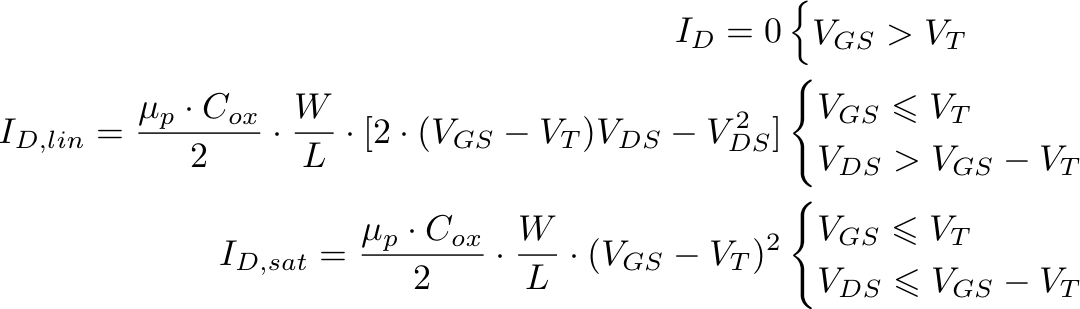## p-channel MOSFET

The equations for the drain current of a p-channel MOSFET in cut-off, linear and saturation mode are:Here ID is the drain current, VDS is the drain-source voltage, VGS is the gate-source voltage, VT is the threshold voltage, L is the length of the transistor, W is the width of the transistor, Cox is the specific capacitance of the gate in F/m², and μp is the mobility.

MOSFET charateristics are typically drawn for serveral gate voltages. In the figure below, the threshold voltage is -0.7 V and the gate voltages are -2, -3, -4, -5 and -6 V.

 IDS [mA] 01234567891001234567 VDS [V]
 W = m L = m Cox = F/m² μp = cm²/Vs VT = V VDS,min = V Vg = V Vg = V Vg = V Vg = V Vg = V Vg = V Vg = V Vg = V Vg = V Vg = V